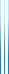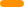# PublicationsPapers

## Twenty (simple) questions

Yuval Dagan, Yuval Filmus, Ariel Gabizon and Shay Moran
STOC 2017, Invited to HALG 2018

Given a distribution $\mu$, the goal of the distributional 20 questions game is to construct a strategy that identifies an unknown element drawn from $\mu$ using as little yes/no queries on average. Huffman’s algorithm constructs an optimal strategy, but the questions one has to ask can be arbitrary.

Given a parameter $n$, we ask how large a set of questions $Q$ needs to be so that for each distribution supported on $[n]$ there is a good strategy which uses only questions from $Q$.

Our first major result is that a linear number of questions (corresponding to binary comparison search trees) suffices to recover the $H(\mu)+1$ performance of Huffman’s algorithm. As a corollary, we deduce that the number of questions needed to guarantee a cost of at most $H(\mu)+r$ (for integer $r$) is asymptotic to $rn^{1/r}$.

Our second major result is that (roughly) $1.25^n$ questions are sufficient to match the performance of Huffman’s algorithm exactly, and this is tight for infinitely many $n$.

We also determine the number of questions sufficient to match the performance of Huffman’s algorithm up to $r$ to be $\Theta(n^{\Theta(1/r)})$.

The second part has appeared been published in Combinatorica. We hope to publish the first part at some point at an information theory journal.

The full version incorporates a third part (since relegated to a different paper), in which we show that the set of questions used to obtain the bound $H(\mu)+1$ performs better when the maximal probability of $\mu$ is small, bounding the performance between 0.5011 and 0.58607.

The full version also contains an extensive literature review, as well as many open questions.copy to clipboard